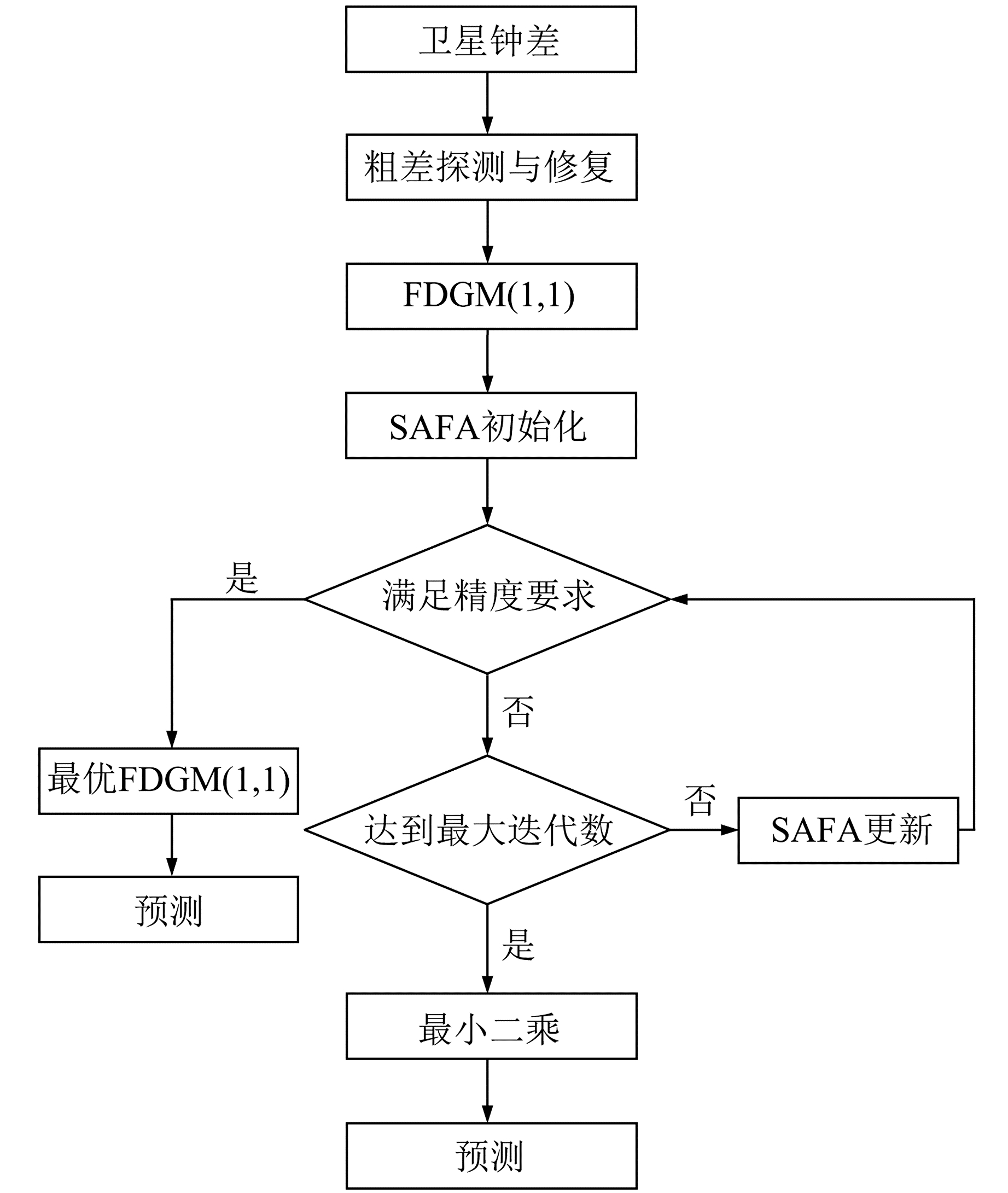﻿ 基于SAFA-FDGM(1, 1)模型的BDS钟差预报文章快速检索 高级检索
 大地测量与地球动力学2021, Vol. 41Issue (7): 672-675  DOI: 10.14075/j.jgg.2021.07.003### 引用本文YUAN Debao, ZHANG Jian, ZHANG Zhenchao, et al. BDS Clock Error Prediction Based on SAFA-FDGM(1, 1) Model[J]. Journal of Geodesy and Geodynamics, 2021, 41(7): 672-675.### Foundation support

National Natural Science Foundation of China, No.51474217; The Joint Fund of Natural Science Foundation of Hebei Province Ecological Wisdom Mine, No.E2020402086.

### 第一作者简介

YUAN Debao, PhD, associate professor, majors in GNSS data processing and deformation monitoring, E-mail: yuandb@cumtb.edu.cn.

### 文章历史

1. 中国矿业大学(北京)地球科学与测绘工程学院，北京市学院路丁11号，100083;
2. 山东科技大学测绘与空间信息学院，青岛市前湾港路579号，266590

1 分数阶离散型灰色系统模型FDGM (1, 1)

 ${\hat x^{(r)}}(k + 1) = {\beta _1}{\hat x^{(r)}}(k) + {\beta _2}$ (1)

2 SAFA-FDGM(1, 1)模型图 1 基于SAFA-FDGM(1, 1)的钟差预报流程 Fig. 1 Flow chart of clock error prediction based on SAFA-FDGM(1, 1)
2.1 改进的萤火虫算法(SAFA)模型

 ${w_i}(t) = \left\{ {\begin{array}{*{20}{l}} {0.9 - 0.5 \times \frac{1}{{1 + {{\rm{e}}^{3.4 - 0.07t}}}}, {I_i} > {I_{{\rm{avg}}}}}\\ {0.9, {I_i} \le {I_{{\rm{avg}}}}} \end{array}} \right.$ (2)

 $\begin{array}{l} {x_i}(t + 1) = {\rm{ }}{w_i}(t){x_i}(t) + \beta ({x_j}(t) - \\ \;\;\;\;\;\;\;{x_i}(t)) + \alpha ({\rm{rand}} - 0.5) \end{array}$ (3)

2.2 仿真实验分析表 1 各算法30次寻优结果的均方根误差 Tab. 1 The root mean square error of 30 optimization results of each algorithm
2.3 基于SAFA-FDGM(1, 1)模型的BDS钟差预报

1) 对BDS钟差数据进行预处理，去除粗差数据，并通过插值算法修复其异常值。

2) 设BDS原始观测钟差序列为X(0)={X(0)(1), X(0)(2), …, X(0)(n)}，通过历元差分降低BDS钟差数据的数量级，提高模型预报精度，即

 $\Delta {X^{(0)}} = \left\{ {\Delta {X^{(0)}}(1), \Delta {X^{(0)}}(2), \cdots , \Delta {X^{(0)}}(n - 1)} \right\}$ (4)

3) 基于差分拟合数据均方根误差最小准则，将分数阶离散型灰色系统模型的阶数作为萤火虫个体，利用SAFA算法优化获取分数阶离散型灰色系统模型(FDGM(1, 1))的最优阶数r

4) 根据最优阶数r确定FDGM(1, 1)模型结构，进行BDS钟差预报。

3 实验分析 3.1 数据来源

3.2 建模方案

3.3 结果分析表 2 各模型3 h预报精度评估 Tab. 2 Evaluation of 3 h forecast accuracy of each model表 3 各模型6 h预报精度评估 Tab. 3 Evaluation of 6 h forecast accuracy of each model表 4 各模型9 h预报精度评估 Tab. 4 Evaluation of 9 h forecast accuracy of each model表 5 各模型12 h预报精度评估 Tab. 5 Evaluation of 12 h forecast accuracy of each model

4 结语

  于烨, 张慧君, 李孝辉, 等. 基于Vondrák滤波1阶差分灰色模型的卫星钟差预报方法[J]. 天文学报, 2018, 59(2): 73-82 (Yu Ye, Zhang Huijun, Li Xiaohui, et al. Prediction Method of Satellite Clock Bias Based on Grey Model of First-Order Difference of Vondrák Filter[J]. Acta Astronomica Sinica, 2018, 59(2): 73-82) (0)  宫晓春, 王宇谱, 王宁, 等. GPS卫星钟差改正数实时预报算法[J]. 武汉大学学报: 信息科学版, 2018, 43(6): 867-873 (Gong Xiaochun, Wang Yupu, Wang Ning, et al. Real-Time Prediction of GPS Satellite Clock Bias Correction[J]. Geomatics and Information Science of Wuhan University, 2018, 43(6): 867-873) (0)  王宇谱, 陈正生, 李伟杰, 等. BDS卫星钟差短期预报性能分析[J]. 大地测量与地球动力学, 2017, 37(5): 450-456 (Wang Yupu, Chen Zhengsheng, Li Weijie, et al. Analyzing the Short-Term Prediction Performance of BDS Satellite Clock Bias[J]. Journal of Geodesy and Geodynamics, 2017, 37(5): 450-456) (0)  于烨, 黄默, 王小青, 等. 利用最小一乘法改进的灰色模型的导航卫星钟差预报[J]. 测绘通报, 2019(4): 1-6 (Yu Ye, Huang Mo, Wang Xiaoqing, et al. Navigation Satellite Clock Bias Prediction Based on Grey Model Improved by Least Absolute Deviations[J]. Bulletin of Surveying and Mapping, 2019(4): 1-6) (0)  李成龙, 陈西宏, 刘继业, 等. 利用自适应TS-IPSO优化的灰色系统预报卫星钟差[J]. 武汉大学学报: 信息科学版, 2018, 43(6): 854-859 (Li Chenglong, Chen Xihong, Liu Jiye, et al. Predicting Satellite Clock Errors Using Grey Model Optimized by Adaptive TS-IPSO[J]. Geomatics and Information Science of Wuhan University, 2018, 43(6): 854-859) (0)  雷雨, 赵丹宁, 蔡宏兵. 利用结构自适应极端学习机预报导航卫星钟差[J]. 武汉大学学报: 信息科学版, 2018, 43(5): 664-668 (Lei Yu, Zhao Danning, Cai Hongbing. Prediction of Navigation Satellite Clock Offset by Adaptive Extreme Learning Machine[J]. Geomatics and Information Science of Wuhan University, 2018, 43(5): 664-668) (0)  陈演羽, 李廷会, 黄飞江, 等. 基于混沌时间序列的GPS卫星钟差预测算法[J]. 仪器仪表学报, 2018, 39(4): 115-122 (Chen Yanyu, Li Tinghui, Huang Feijiang, et al. GPS Satellite Clock Bias Prediction Algorithm Based on Chaotic Time Series[J]. Chinese Journal of Scientific Instrument, 2018, 39(4): 115-122) (0)  王利, 张勤, 黄观文, 等. 基于指数平滑法的GPS卫星钟差预报[J]. 武汉大学学报: 信息科学版, 2017, 42(7): 995-1001 (Wang Li, Zhang Qin, Huang Guanwen, et al. GPS Satellite Clock Bias Prediction Based on Exponential Smoothing Method[J]. Geomatics and Information Science of Wuhan University, 2017, 42(7): 995-1001) (0)  李源, 战兴群, 梅浩, 等. 灰色粒子群自适应卫星钟差预报方法[J]. 哈尔滨工业大学学报, 2018, 50(4): 71-77 (Li Yuan, Zhan Xingqun, Mei Hao, et al. Particle Swarm Adaptive Satellite Clock Error Prediction Model Based on Grey Theory[J]. Journal of Harbin Institute of Technology, 2018, 50(4): 71-77) (0)  吴利丰, 刘思峰, 姚立根. 基于分数阶累加的离散灰色模型[J]. 系统工程理论与实践, 2014, 34(7): 1822-1827 (Wu Lifeng, Liu Sifeng, Yao Ligen. Discrete Grey Model Based on Fractional Order Accumulate[J]. Systems Engineering——Theory and Practice, 2014, 34(7): 1822-1827) (0)  Yang X S. Nature-Inspired Metaheuristic Algorithms[M]. London: Luniver Press, 2008 (0)  Yang X S. Firefly Algorithms for Multimodal Optimization[C]. Proceedings of the 5th International Conference on Stochastic Algorithms: Foundations and Applications, Heidelberg, 2009 (0)  汪靖, 刘桂元. 基于动态步长变化的萤火虫算法[J]. 计算机工程与设计, 2019, 40(4): 1001-1007 (Wang Jing, Liu Guiyuan. Improved Firefly Algorithm Based on Dynamic Step[J]. Computer Engineering and Design, 2019, 40(4): 1001-1007) (0)  黄洋, 鲁海燕, 许凯波, 等. 基于S型函数的自适应粒子群优化算法[J]. 计算机科学, 2019, 46(1): 245-250 (Huang Yang, Lu Haiyan, Xu Kaibo, et al. S-Shaped Function Based Adaptive Particle Swarm Optimization Algorithm[J]. Computer Science, 2019, 46(1): 245-250) (0)
BDS Clock Error Prediction Based on SAFA-FDGM(1, 1) Model
YUAN Debao1ZHANG Jian1     ZHANG Zhenchao1     WEI Shengtao2
1. College of Geoscience and Surveying Engineering, China University of Mining and Technology, D11 Xueyuan Road, Beijing 100083, China;
2. College of Geodesy and Geomatics, Shandong University of Science and Technology, 579 Qianwangang Road, Qingdao 266590, China
Abstract: In order to establish a high-precision BDS clock error prediction model, we propose a SAFA-FDGM(1, 1) clock error prediction model based on the fractional-order discrete gray system optimized by the improved firefly algorithm. In order to avoid the firefly algorithm falling into the local optimal solution, and improve the firefly algorithm optimization ability. We introduce the inertia weight factor and improve the attractiveness factor and step factor, and use the improved firefly algorithm to automatically optimize the selection of the fractional-order factor of FDGM(1, 1) model to improve the accuracy of FDGM(1, 1) data fitting. We apply the clock error data of three different types of satellites, C02(GEO), C09(IGSO) and C12(MEO), for test analysis. The results show that the proposed clock error prediction model is superior to the traditional quadratic polynomial model and the GM(1, 1) model, 3~6 h prediction error is less than 1 ns, and the 9~12 h prediction error is better than 2 ns, which has important reference value to establish BDS satellite universal clock error prediction model.
Key words: BDS; clock error prediction model; improved firefly algorithm; FDGM(1, 1)